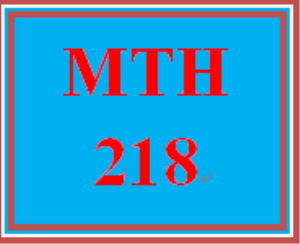MTH 218 Week 3 Signature Assignment Stage 2: Calculations and Visuals

PLDZ-5704 In Stock
\$ 8.00 USD
Description

MTH 218 Week 3 Signature Assignment Stage 2: Calculations and Visuals

https://zh.scribd.com/document/343514419/Uop-Tutorial

MTH 218 Week 3 Signature Assignment Stage 2: Calculations and Visuals

In this stage, you will do statistical reasoning and mathematical modeling to show central tendency and two variable analyses, including regression with equation and R squared value.

Create at least three visuals:

Required: One visual must be a scatterplot with trend line, equation and R square value.

Optional: Histograms, box and whisker plots, or frequency tables.

Note: depending on your topic and question you may want to use your data to calculate probabilities.

Create a word document that includes your three visuals and the following items:

• Brief description of your visuals.

• Show visuals, including at least one graph that is an xy scatterplot with trend line, equation, and R square value. Make sure the data satisfies the following criteria:

• The inputs and outputs are quantitative data that consist of an independent and dependent variable. Ideal data for this graph would consist of inputs that are years and outputs that consist of values associated with particular years.

• The data should have central relevance to your topic. Make sure the data supports your overall conclusion.

• Make sure your visuals have:

• A title (consider the W’s – who, what, where, why and when).

• A legend (if applicable)

• For your xy scatterplotmake at least one prediction using the trend line equationfor some date into the future. Show your calculations to the group. How confident are you in this prediction? State your prediction and provide justification.

• If applicable, calculate the mean and standard deviation of the sample data. Show the calculations to the group. Show the distribution of the numbers in a histogram.  Describe the shape of the plot and the spread of the numbers.

• If applicable, calculate probabilities based on your data.  How do these probabilities support your argument?

• If applicable, create a box and whisker plot. Describe the central tendency of the values. What does this tell you about the data? About your project?

Click the Assignment Files tab to submit your assignment.

Recent Reviews Write a Review
0 0 0 0 reviews